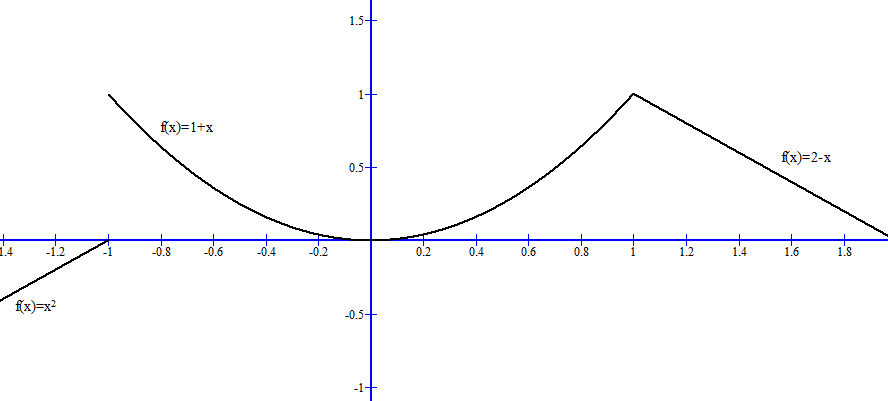×

×

# Solved: Sketch the graph of the function and use it to determine the values of a forISBN: 9781305270336 484

## Solution for problem 11 Chapter 2.2

Single Variable Calculus: Early Transcendentals | 8th Edition

• Textbook Solutions
• 2901 Step-by-step solutions solved by professors and subject experts
• Get 24/7 help from StudySoup virtual teaching assistantsSingle Variable Calculus: Early Transcendentals | 8th Edition

4 5 1 272 Reviews
12
5
Problem 11

Sketch the graph of the function and use it to determine the values of a for which limxla fsxd exists. fsxd H 1 1 x x 2 2 2 x if x , 21 if 21 < x , 1 if x > 1

Step-by-Step Solution:

Problem 11

Sketch the graph of the function and use it to determine the value of a for whichexistsStep by Step Solution

Step 1 of 3

Given function isTo sketch the function f(x).Step 2 of 3

Step 3 of 3

##### ISBN: 9781305270336

Since the solution to 11 from 2.2 chapter was answered, more than 279 students have viewed the full step-by-step answer. The full step-by-step solution to problem: 11 from chapter: 2.2 was answered by , our top Calculus solution expert on 03/19/18, 03:29PM. This full solution covers the following key subjects: . This expansive textbook survival guide covers 95 chapters, and 5427 solutions. This textbook survival guide was created for the textbook: Single Variable Calculus: Early Transcendentals, edition: 8. Single Variable Calculus: Early Transcendentals was written by and is associated to the ISBN: 9781305270336. The answer to “Sketch the graph of the function and use it to determine the values of a for which limxla fsxd exists. fsxd H 1 1 x x 2 2 2 x if x , 21 if 21 < x , 1 if x > 1” is broken down into a number of easy to follow steps, and 44 words.

Unlock Textbook Solution